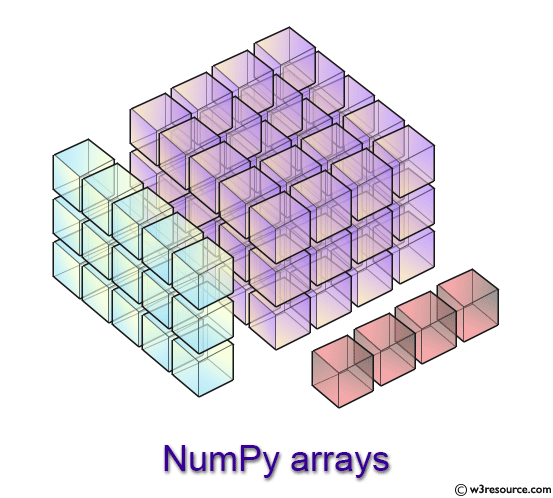# NumPy: Array manipulation routines

## Array manipulation routines

This section present the functions of Basic operations, Changing array shape, Transpose-like operations, Changing number of dimensions, Changing kind of array, Joining arrays, Splitting arrays, Tiling arrays, Adding and removing elements and Rearranging elements to access data and subarrays, and to split, reshape, and join the arrays.Basic operations Name Description Syntax copyto() Return a new array of given shape and type, without initializing entries. empty(shape[, dtype, order])
 Changing array shape Name Description Syntax reshape() Gives a new shape to an array without changing its data. reshape(a, newshape[, order]) ravel() Return a contiguous flattened array. ravel(a[, order]) ndarray.flat A 1-D iterator over the array. ndarray.flat ndarray.flatten() Return a copy of the array collapsed into one dimension. ndarray.flatten([order])
 Transpose-like operations Name Description Syntax moveaxis() Move axes of an array to new positions. moveaxis(a, source, destination) rollaxis Roll the specified axis backwards, until it lies in a given position. rollaxis(a, axis[, start]) swapaxes() Interchange two axes of an array. swapaxes(a, axis1, axis2) ndarray.T Same as self.transpose(), except that self is returned if self.ndim < 2. transpose() Return a full array with the same shape and type as a given array. full_like(a, fill_value[, dtype, order, subok])
 Changing number of dimensions Name Description Syntax atleast_1d() Convert inputs to arrays with at least one dimension. atleast_1d(*arys) atleast_2d() View inputs as arrays with at least two dimensions. atleast_2d(*arys) atleast_3d() View inputs as arrays with at least three dimensions. atleast_3d(*arys) broadcast Produce an object that mimics broadcasting. broadcast broadcast_to() Broadcast an array to a new shape. broadcast_to(array, shape[, subok]) broadcast_arrays() Broadcast any number of arrays against each other. broadcast_arrays(*args, **kwargs) expand_dims() Expand the shape of an array. expand_dims(a, axis) squeeze() Remove single-dimensional entries from the shape of an array. squeeze(a[, axis])
 Changing kind of array Name Description Syntax asarray() Convert the input to an array. asarray(a[, dtype, order]) asanyarray() Convert the input to an ndarray, but pass ndarray subclasses through. asanyarray(a[, dtype, order]) asmatrix() Interpret the input as a matrix. asmatrix(data[, dtype]) asfarray() Return an array converted to a float type. asfarray(a[, dtype]) asfortranarray() Return an array laid out in Fortran order in memory. asfortranarray(a[, dtype]) ascontiguousarray() Return a contiguous array in memory (C order). ascontiguousarray(a[, dtype]) asarray_chkfinite() Convert the input to an array, checking for NaNs or Infs. asarray_chkfinite(a[, dtype, order]) asscalar Convert an array of size 1 to its scalar equivalent. asscalar(a) require Return an ndarray of the provided type that satisfies requirements. require(a[, dtype, requirements])
 Joining arrays Name Description Syntax concatenate() Join a sequence of arrays along an existing axis. concatenate((a1, a2, …)[, axis, out]) stack() Join a sequence of arrays along a new axis. stack(arrays[, axis, out]) column_stack() Stack 1-D arrays as columns into a 2-D array. column_stack(tup) dstack() Stack arrays in sequence depth wise (along third axis). dstack(tup) hstack() Stack arrays in sequence horizontally (column wise). hstack(tup) vstack() Stack arrays in sequence vertically (row wise). vstack(tup) block() Assemble an nd-array from nested lists of blocks. block(arrays)
 Splitting arrays Name Description Syntax split() Split an array into multiple sub-arrays. split(ary, indices_or_sections[, axis]) array_split() Split an array into multiple sub-arrays. array_split(ary, indices_or_sections[, axis]) dsplit() Split array into multiple sub-arrays along the 3rd axis (depth). dsplit(ary, indices_or_sections) hsplit() Split an array into multiple sub-arrays horizontally (column-wise). hsplit(ary, indices_or_sections) vsplit() Split an array into multiple sub-arrays vertically (row-wise). vsplit(ary, indices_or_sections)
 Tiling arrays Name Description Syntax tile() Construct an array by repeating A the number of times given by reps. tile(A, reps) repeat() Repeat elements of an array. repeat(a, repeats[, axis])
 Adding and removing elements Name Description Syntax delete() Return a new array with sub-arrays along an axis deleted. delete(arr, obj[, axis]) insert() Insert values along the given axis before the given indices. insert(arr, obj, values[, axis]) append() Append values to the end of an array. append(arr, values[, axis]) resize() Return a new array with the specified shape. resize(a, new_shape) trim_zeros() Trim the leading and/or trailing zeros from a 1-D array or sequence. trim_zeros(filt[, trim]) unique() Find the unique elements of an array. unique(ar[, return_index, return_inverse, …])
 Rearranging elements Name Description Syntax flip() Reverse the order of elements in an array along the given axis. flip(m[, axis]) fliplr() Flip array in the left/right direction. fliplr(m) flipud() Flip array in the up/down direction. flipud(m) reshape() Gives a new shape to an array without changing its data. reshape(a, newshape[, order]) roll() Roll array elements along a given axis. roll(a, shift[, axis]) rot90() Rotate an array by 90 degrees in the plane specified by axes. rot90(m[, k, axes]))

Previous: bmat()
Next: Basic operations copyto()

﻿